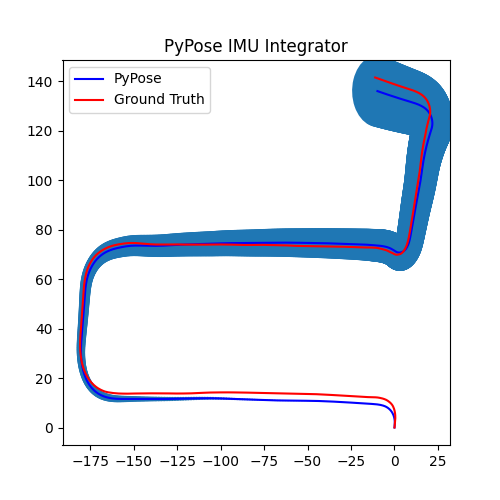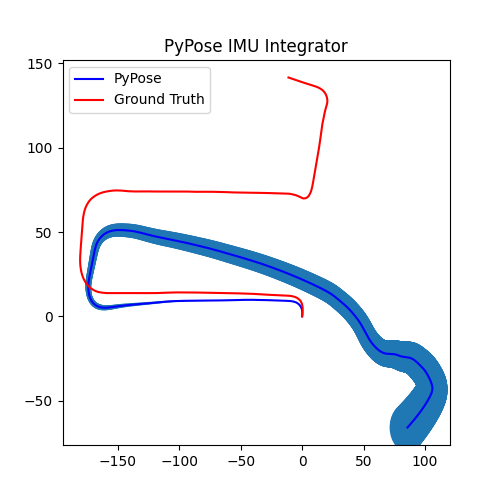Shortcuts

# pypose.module.IMUPreintegrator¶

class pypose.module.IMUPreintegrator(pos=tensor([0., 0., 0.]), rot=SO3Type LieTensor: LieTensor([0., 0., 0., 1.]), vel=tensor([0., 0., 0.]), gravity=9.81007, gyro_cov=1.024e-05, acc_cov=0.0064, prop_cov=True, reset=False)[source]

Applies preintegration over IMU input signals.

Parameters
• pos (torch.Tensor, optional) – initial position. Default: torch.zeros(3).

• rot (pypose.SO3, optional) – initial rotation. Default: pypose.identity_SO3().

• vel (torch.Tensor, optional) – initial position. Default: torch.zeros(3).

• gravity (float, optional) – the gravity acceleration. Default: 9.81007.

• gyro_cov – covariance of the gyroscope. Default: (3.2e-3)**2. Use the form torch.Tensor(gyro_cov_x, gyro_cov_y, gyro_cov_z), if the covariance on the three axes are different.

• acc_cov – covariance of the accelerator. Default: (8e-2)**2. Use the form torch.Tensor(acc_cov_x, acc_cov_y,acc_cov_z), if the covariance on the three axes are different.

• prop_cov (Bool, optional) – flag to propagate the covariance matrix. Default: True.

• reset (Bool, optional) – flag to reset the initial states after the forward function is called. If False, the integration starts from the last integration. This flag is ignored if init_state is not None. Default: False.

forward(dt, gyro, acc, rot=None, gyro_cov=None, acc_cov=None, init_state=None)[source]

Propagate IMU states from duration ($$\delta t$$), gyroscope (angular rate $$\omega$$), linear acceleration ($$\mathbf{a}$$) in body frame, as well as their measurement covariance for gyroscope $$C_{g}$$ and acceleration $$C_{\mathbf{a}}$$. Known IMU rotation estimation $$R$$ can be provided for better precision.

Parameters
• dt (torch.Tensor) – time interval from last update.

• gyro (torch.Tensor) – angular rate ($$\omega$$) in IMU body frame.

• acc (torch.Tensor) – linear acceleration ($$\mathbf{a}$$) in IMU body frame (raw sensor input with gravity).

• rot (pypose.SO3, optional) – known IMU rotation.

• gyro_cov (torch.Tensor, optional) – covariance matrix of angular rate. If not given, the default state in constructor will be used.

• acc_cov (torch.Tensor, optional) – covariance matrix of linear acceleration. If not given, the default state in constructor will be used.

• init_state (Dict, optional) – the initial state of the integration. The dictionary should be in form of {'pos': torch.Tensor, 'rot': pypose.SO3, 'vel': torch.Tensor}. If not given, the initial state in constructor will be used.

Shape:
• input (dt, gyro, acc): This layer supports the input shape with $$(B, F, H_{in})$$, $$(F, H_{in})$$ and $$(H_{in})$$, where $$B$$ is the batch size (or the number of IMU), $$F$$ is the number of frames (measurements), and $$H_{in}$$ is the raw sensor signals.

• init_state (Optional): The initial state of the integration. It contains pos: initial position, rot: initial rotation, vel: initial velocity, with the shape $$(B, H_{in})$$

• output: a dict of integrated state including pos: position, rot: rotation, and vel: velocity, each of which has a shape $$(B, F, H_{out})$$, where $$H_{out}$$ is the signal dimension. If prop_cov is True, it will also include cov: covariance matrix in shape of $$(B, 9, 9)$$.

IMU Measurements Integration:

\begin{align*} {\Delta}R_{ik+1} &= {\Delta}R_{ik} \mathrm{Exp} (w_k {\Delta}t) \\ {\Delta}v_{ik+1} &= {\Delta}v_{ik} + {\Delta}R_{ik} a_k {\Delta}t \\ {\Delta}p_{ik+1} &= {\Delta}p_{ik} + {\Delta}v_{ik} {\Delta}t + \frac{1}{2} {\Delta}R_{ik} a_k {\Delta}t^2 \end{align*}

where:

• $${\Delta}R_{ik}$$ is the preintegrated rotation between the $$i$$-th and $$k$$-th time step.

• $${\Delta}v_{ik}$$ is the preintegrated velocity between the $$i$$-th and $$k$$-th time step.

• $${\Delta}p_{ik}$$ is the preintegrated position between the $$i$$-th and $$k$$-th time step.

• $$a_k$$ is linear acceleration at the $$k$$-th time step.

• $$w_k$$ is angular rate at the $$k$$-th time step.

• $${\Delta}t$$ is the time interval from time step $$k$$-th to time step $${k+1}$$-th time step.

Uncertainty Propagation:

\begin{align*} C_{ik+1} &= A C_{ik} A^T + B \mathrm{diag}(C_g, C_a) B^T \\ &= A C A^T + B_g C_g B_g^T + B_a C_a B_a^T \end{align*},

where

$A = \begin{bmatrix} {\Delta}R_{ik+1}^T & 0_{3*3} \\ -{\Delta}R_{ik} (a_k^{\wedge}) {\Delta}t & I_{3*3} & 0_{3*3} \\ -1/2{\Delta}R_{ik} (a_k^{\wedge}) {\Delta}t^2 & I_{3*3} {\Delta}t & I_{3*3} \end{bmatrix},$
$B = [B_g, B_a] \\$
$B_g = \begin{bmatrix} J_r^k \Delta t \\ 0_{3*3} \\ 0_{3*3} \end{bmatrix}, B_a = \begin{bmatrix} 0_{3*3} \\ {\Delta}R_{ik} {\Delta}t \\ 1/2 {\Delta}R_{ik} {\Delta}t^2 \end{bmatrix},$

where $$\cdot^\wedge$$ is the skew matrix (pypose.vec2skew()), $$C \in\mathbf{R}^{9\times 9}$$ is the covariance matrix, and $$J_r^k$$ is the right jacobian (pypose.Jr()) of integrated rotation $$\mathrm{Exp}(w_k{\Delta}t)$$ at $$k$$-th time step, $$C_{g}$$ and $$C_{\mathbf{a}}$$ are measurement covariance of angular rate and acceleration, respectively.

Note

Output covariance (Shape: $$(B, 9, 9)$$) is in the order of rotation, velocity, and position.

With IMU preintegration, the propagated IMU status:

\begin{align*} R_j &= {\Delta}R_{ij} * R_i \\ v_j &= R_i * {\Delta}v_{ij} + v_i + g \Delta t_{ij} \\ p_j &= R_i * {\Delta}p_{ij} + p_i + v_i \Delta t_{ij} + 1/2 g \Delta t_{ij}^2 \\ \end{align*}

where:

• $${\Delta}R_{ij}$$, $${\Delta}v_{ij}$$, $${\Delta}p_{ij}$$ are the preintegrated measurements.

• $$R_i$$, $$v_i$$, and $$p_i$$ are the initial state. Default initial values are used if reset is True.

• $$R_j$$, $$v_j$$, and $$p_j$$ are the propagated state variables.

• $${\Delta}t_{ij}$$ is the time interval from frame i to j.

Note

The implementation is based on Eq. (A7), (A8), (A9), and (A10) of this report:

Example

1. Preintegrator Initialization

>>> import torch
>>> import pypose as pp
>>> p = torch.zeros(3)    # Initial Position
>>> r = pp.identity_SO3() # Initial rotation
>>> v = torch.zeros(3)    # Initial Velocity
>>> integrator = pp.module.IMUPreintegrator(p, r, v)

1. Get IMU measurement

>>> ang = torch.tensor([0.1,0.1,0.1]) # angular velocity
>>> acc = torch.tensor([0.1,0.1,0.1]) # acceleration
>>> rot = pp.mat2SO3(torch.eye(3))    # Rotation (Optional)
>>> dt = torch.tensor([0.002])        # Time difference between two measurements


3. Preintegrating IMU measurements. Takes as input the IMU values and calculates the preintegrated IMU measurements.

>>> states = integrator(dt, ang, acc, rot)
{'rot': SO3Type LieTensor:
tensor([[[1.0000e-04, 1.0000e-04, 1.0000e-04, 1.0000e+00]]]),
'vel': tensor([[[ 0.0002,  0.0002, -0.0194]]]),
'pos': tensor([[[ 2.0000e-07,  2.0000e-07, -1.9420e-05]]]),
'cov': tensor([[[ 5.7583e-11, -5.6826e-19, -5.6827e-19,  0.0000e+00,  0.0000e+00,
0.0000e+00,  0.0000e+00,  0.0000e+00,  0.0000e+00],
[-5.6826e-19,  5.7583e-11, -5.6827e-19,  0.0000e+00,  0.0000e+00,
0.0000e+00,  0.0000e+00,  0.0000e+00,  0.0000e+00],
[-5.6827e-19, -5.6827e-19,  5.7583e-11,  0.0000e+00,  0.0000e+00,
0.0000e+00,  0.0000e+00,  0.0000e+00,  0.0000e+00],
[ 0.0000e+00,  0.0000e+00,  0.0000e+00,  8.0000e-09, -3.3346e-20,
-1.0588e-19,  8.0000e-12,  1.5424e-23, -1.0340e-22],
[ 0.0000e+00,  0.0000e+00,  0.0000e+00, -1.3922e-19,  8.0000e-09,
0.0000e+00, -8.7974e-23,  8.0000e-12,  0.0000e+00],
[ 0.0000e+00,  0.0000e+00,  0.0000e+00,  0.0000e+00, -1.0588e-19,
8.0000e-09,  0.0000e+00, -1.0340e-22,  8.0000e-12],
[ 0.0000e+00,  0.0000e+00,  0.0000e+00,  8.0000e-12,  1.5424e-23,
-1.0340e-22,  8.0000e-15, -1.2868e-26,  0.0000e+00],
[ 0.0000e+00,  0.0000e+00,  0.0000e+00, -8.7974e-23,  8.0000e-12,
0.0000e+00, -1.2868e-26,  8.0000e-15,  0.0000e+00],
[ 0.0000e+00,  0.0000e+00,  0.0000e+00,  0.0000e+00, -1.0340e-22,
8.0000e-12,  0.0000e+00,  0.0000e+00,  8.0000e-15]]])}


Preintegrated IMU odometry from the KITTI dataset with and without known rotation.Fig. 1. Known Rotation.Fig. 2. Estimated Rotation.

Note

The examples generating the above figures can be found at examples/module/imu.## Docs

Access documentation for PyPose

View Docs

## Tutorials

Get started with tutorials and examples

View Tutorials

## Get Started

Find resources and how to start using pypose

View Resources